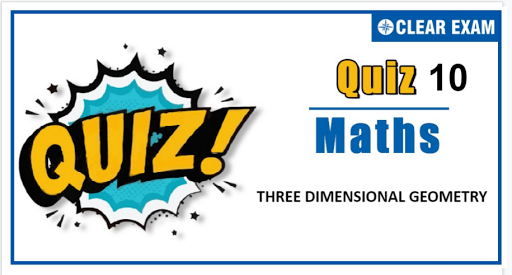## Three Dimensional Geometry Quiz

Important topics for Maths has been designed in such a way that it offers very practical and application-based learning to further make it easier for students to understand every concept or topic by correlating it with the day-to-day experiences.

Q1. The shortest distance from the plane 12x+y+3z=327 to the sphere x^2+y^2+z^2+4x-2y-6z=155 is
•  39
•  26
•  41 4/13
•  13
Q2.The angle between i ̂ line of the intersection of the plane r ∙(i ̂+2j ̂+3k ̂ )=0 and r ∙(3i ̂+3j ̂+k ̂ )=0, is
•  cos^(-1)⁡(1/3)
•  cos^(-1)⁡(1/√3)
•  cos^(-1)⁡(2/√3)
•  None of these
Solution
(d) Line of intersection of r ∙(i ̂+2j ̂+3k ̂ )=0 and r ∙(3i ̂+3j ̂+k ̂ )=0 will be parallel to (3i ̂+3j ̂+k ̂ )×(i ̂+2j ̂+3k ̂ ), i.e., 7i ̂-8j ̂+3k ̂ If the required angle is θ, then cos⁡θ=7/√(49+64+9)=7/√122

Q4. Let PM be the perpendicular from the point P(1,2,3) to the x-y plane. If (OP) makes an angle θ with the positive direction of the z-axis and (OM) makes an angle ϕ with the positive direction of x-axis, where O is the origin and θ and ϕ are acute angles, then
•  cos⁡θ cos⁡ϕ=1/√14
•  sin⁡θ sin⁡ϕ=2/√14
•  tan⁡ϕ=2
•  tan⁡θ=√5/3
Solution
(d) If P be (x,y,z), then from the figure x=r sin⁡θ cos⁡ϕ,y=r sin⁡θ sin⁡ϕ and z=r cos⁡θ 1=r sin⁡θ cos⁡ϕ,2=r sin⁡θ sin⁡ϕ and 3=r cos⁡θ ⇒1^2+2^2+3^2=r^2 ⇒r=±√14 ∴ sin⁡θ cos⁡ϕ=1/√14,sin⁡θ sin⁡ϕ=2/√14 and cos⁡θ=3/√14 (neglecting negative sign as θ and ϕ are acute) (sin⁡θ sin⁡ϕ)/(sin⁡θ cos⁡ϕ )=2/1 ⇒tan⁡ϕ=2 Also, tan⁡θ=√5/3

Q5.The equation of the line x+y+z-1=0 and 4x+y-2z+2=0 written in the symmetrical form is
•  (x-1)/2=(y+2)/(-1)=(z-2)/2
•  (x+(1/2))/1=(y-1)/(-2)=(z-(1/2))/1
•  x/1=y/(-2)=(z-1)/1
•  (x+1)/1=(y-2)/(-2)=(z-0)/1
Solution
(b) x+y+z-1=0 4x+y-2z+2=0 Therefore, the line is along the vector (i ̂+j ̂+k ̂ )×(4i ̂+j ̂-2k ̂ )=3i ̂-6j ̂+3k ̂ Let z=k. Then x=k-1 and y=2-2k Therefore, (k-1,2-2k,k) is any point on the line Hence, (-1,2,0),(0,0,1) and (-1/2,1,1/2) are the points on the line

Q6. Consider a set of points R in the space which is at a distance of 2 units from the line x/1=(y-1)/(-1)=(z+2)/2 between the planes x-y+2z+3=0 and x-y+2z-2=0
•  The volume of the bounded figure by points R and the planes is (10/3√3)π cube units
•  The area of the curved surface formed by the set of points R is (20π/√6) sq. units
• The volume of the bounded figure by the set of points R and the planes is (20π/√6) cubic units
•  The area of the curved surface formed by the set of points R is (10/√3)π sq. units
Solution
(b) Distance between the planes is h=5/√6 Also the figure formed is cylinder, whose radius is r=2 units Hence, the volume of the cylinder is πr^2 h=π(2)^2.5/√6=20π/√6 cubic units Also the curved surface area is 2πrh=2π(2).5/√6=20π/√6

Q7. A rod of length 2 units whose one end is (1,0,-1) and other end touches the plane x-2y+2z+4=0, then
•  The rod sweeps the figure whose volume is π cubic units
•  The area of the region which the rod traces on the plane is 2π
•  The length of projection of the rod on the plane is √3 units
•  The centre of the region which the rod traces on the plane is (2/3,2/3,(-5)/3)
Solution
(d) The rod sweeps out the figure which is a cone The distance of point A(1,0,-1) from the plane is (|1-2+4|)/√9=1 unit The slant height l of the cone is 2 units Then the radius of the base of the cone is √(l^2-1)=√(4-1)=√3 Hence, the volume of the cone is π/3 (√3)^2.1=π cubic units Area of the circle on the plane which the of traces is 3π Also, the centre of the circle is Q(x,y,z). Then (x-1)/1=(y-0)/(-2)=(z+1)/2=(-(1-0-2+4))/(1^2+(-2^2)+2^2 ), or Q(x,y,z)≡(2/3,2/3,(-5)/3)

Q8. Consider the planes 3x-6y+2z+5=0 and 4x-12y+3z=3. The plane 67x-162y+47z+44=0 bisects the angle between the given planes which
•  Contains the origin
•  Is acute
•  Is obtuse
•  None of these
Solution
(b) 3x-6y+2z+5=0 (i) -4x+12y-3z+3=0 (ii) Bisectors are (3x-6y+2z+5)/√(9+36+4)=±(-4x+12y-3z+3)/√(16+144+9) The plane which bisects the angle between the planes that contains the origin 13(3x-6y+2z+5)=7(-4x+12y-3z+3) 67x-162y+47z+44=0 (iii) Further, 3×(-4)+(-6)(12)+2×(-3)<0 Hence, the origin lies in acute angle

Q10. If OABC is a tetrahedron such that OA^2+BC^2=OB^2+CA^2=OC^2+AB^2, then
•  OA⊥BC
•  OB⊥CA
•  OC⊥AB
• AB⊥BC#### Written by: AUTHORNAME

AUTHORDESCRIPTION## Want to know more

Please fill in the details below:

## Latest NEET Articles\$type=three\$c=3\$author=hide\$comment=hide\$rm=hide\$date=hide\$snippet=hide

Name

ltr
item
BEST NEET COACHING CENTER | BEST IIT JEE COACHING INSTITUTE | BEST NEET & IIT JEE COACHING: Three-Dimensional-Geometry-Quiz-10
Three-Dimensional-Geometry-Quiz-10